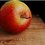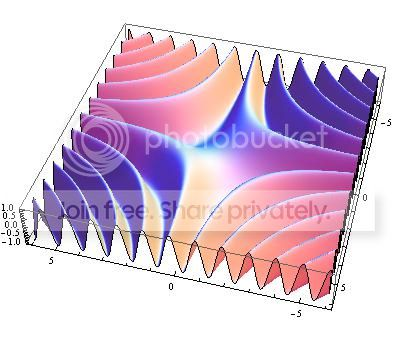# Weird functionshi

Take a look here

This is extremely weird as both functions are algebraically the same. This kind of thing occurs very frequently but it still leaves me puzzled.

What do you think causes the difference when graphing them?Note by Julian Poon
6 years, 8 months ago

This discussion board is a place to discuss our Daily Challenges and the math and science related to those challenges. Explanations are more than just a solution — they should explain the steps and thinking strategies that you used to obtain the solution. Comments should further the discussion of math and science.

When posting on Brilliant:

• Use the emojis to react to an explanation, whether you're congratulating a job well done , or just really confused .
• Ask specific questions about the challenge or the steps in somebody's explanation. Well-posed questions can add a lot to the discussion, but posting "I don't understand!" doesn't help anyone.
• Try to contribute something new to the discussion, whether it is an extension, generalization or other idea related to the challenge.

MarkdownAppears as
*italics* or _italics_ italics
**bold** or __bold__ bold
- bulleted- list
• bulleted
• list
1. numbered2. list
1. numbered
2. list
Note: you must add a full line of space before and after lists for them to show up correctly
paragraph 1paragraph 2

paragraph 1

paragraph 2

[example link](https://brilliant.org)example link
> This is a quote
This is a quote
    # I indented these lines
# 4 spaces, and now they show
# up as a code block.

print "hello world"
# I indented these lines
# 4 spaces, and now they show
# up as a code block.

print "hello world"
MathAppears as
Remember to wrap math in $$ ... $$ or $ ... $ to ensure proper formatting.
2 \times 3 $2 \times 3$
2^{34} $2^{34}$
a_{i-1} $a_{i-1}$
\frac{2}{3} $\frac{2}{3}$
\sqrt{2} $\sqrt{2}$
\sum_{i=1}^3 $\sum_{i=1}^3$
\sin \theta $\sin \theta$
\boxed{123} $\boxed{123}$

Sort by:

In the first place, Sin(xy) is supposed to be a surface, so the green graph sort of looks like the way it's supposed to be (however badly drawn). In theory, both functions are the same, but apparently your graphing calculator has a hangup with the first one. If you use a good math software like Mathematica, there is no difference. Check this outSinXY

- 6 years, 8 months ago

I dont really understand why it is a surface though. $\sin{(xy)}=0$ has only $2$ variables.

- 6 years, 8 months ago

Oh, right, I missed the memo that it has to equal to $0$. Well, you can see where this surface is equal to $0$, so it agrees with the green graph. Let me try something now.

Yeah, I get the same graph as the green one, with both equations you've posted. For whatever reason, your graphing calculator has a hangup with the series expression. Can you give me other examples of this "weird behavior that keeps frequently", and maybe we can see what your calculator is doing?

- 6 years, 8 months ago

Other examples include this

- 6 years, 8 months ago

Well, once again, Mathematica handles this correctly, and shows part of this function $y={ x }^{ 6 }$ in both cases. I think your calculator is just not up to this job. I don't think the reason why it shows the line $x=6$ is related to the problems it's having with the first example you gave.

- 6 years, 8 months ago

May be the functions are non identical.

Looks at these

f(x) = $ln x^{2}$

g(x) = $2lnx$

- 6 years, 8 months ago

Yeah, for a simple case like this the difference can be easily explained. But this one is too complicated and I have no idea what causes this, thats why im posting it.

Nice example by the way :D.

- 6 years, 8 months ago